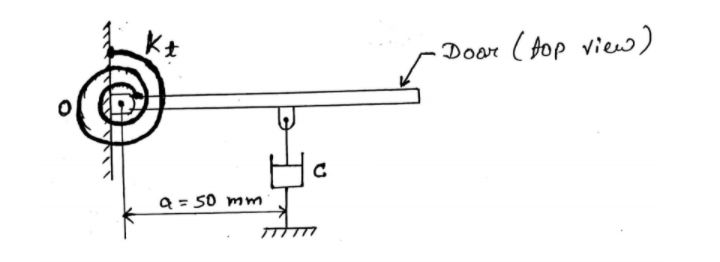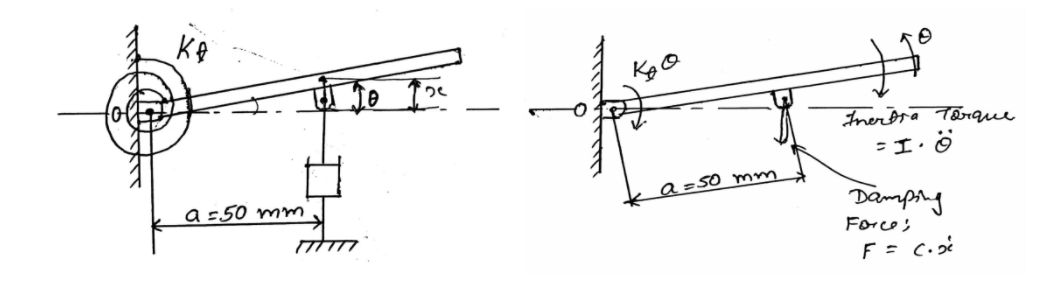# Damped free Vibration - Numerical 4

### Numerical: A door along with door-closing system shown is shown in the figure below. It has a moment of inertia of $25 \ kg \sdot m^2$ about the hinge axis. If the stiffness of torsional spring is $20 \ N m/rad$, find the most suitable value of the damping coefficient.### Solution:Given data:
Moment of inertia, $\ I = 25 \ kg \sdot m^2$
Stiffness of torsional spring $= 20 \ N \sdot m/rad$
$C_c = (?)$

According to D'Alembert's principle;

$\Sigma \bigg[ \ inertia \ torque + \ external \ torque \bigg] = 0$\begin{aligned} \therefore \ I \sdot \ddot \theta + (c_c \dot x) \sdot a + K_t \theta &= 0\\ \therefore \ I \sdot \ddot \theta + c_ca \dot \theta a + K_t \theta &= 0 \quad (x = a \theta \ and \ \dot x = a \dot \theta)\\ \therefore \ I \sdot \ddot \theta + c_ca^2 \dot \theta + K_t \theta &= 0 \end{aligned}

The above equation can be written as:

$\therefore \ I \sdot \ddot \theta \ + c_t \ddot \theta \ + K_t \theta = 0 \quad \quad \text {where} \ \ c_t = c_ca^2$

Now,

\begin{aligned} c_t &= 2 \sdot I \sdot \omega_n\\ \therefore \ c_t &= 2 \sdot \ I \sqrt{K_t \over I}\\ \therefore \ c_c \sdot a^2 &= 2 \sqrt{K_tI} \quad \because (c_t = c_ca^2)\\ \therefore \ c_c &= {2 \over a^2} \sqrt{K_tI}\\ &= {2 \over (0.05)^2} \sqrt{20 \times 25}\\ \therefore \ c_c &= 17888.54 \ N \sdot s/m \end{aligned}